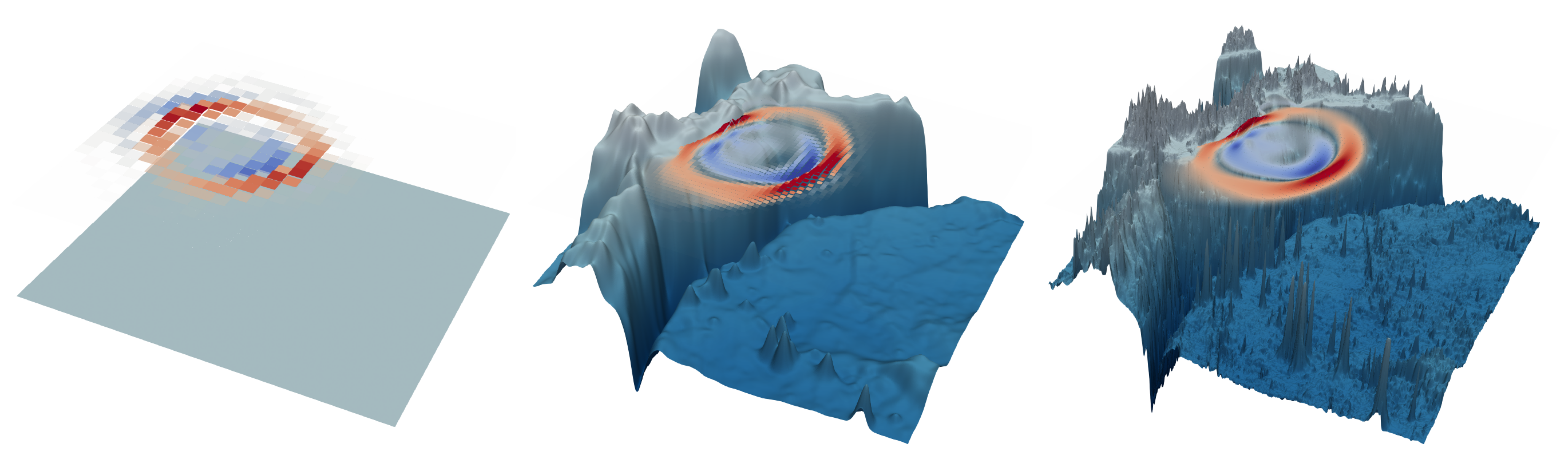# Tsunami¶

## Overview¶

In this benchmark we model the propagation of the 2011 Tohoku tsunami by solving the shallow water equations. For the numerical solution of the PDE, we apply an ADER-DG method implemented in the ExaHyPE framework. The aim is to obtain the parameters describing the initial displacements from the data of two available buoys located near the Japanese coast.## Run¶

docker run -it -p 4242:4242 linusseelinger/model-exahype-tsunami


## Properties¶

Model

Description

forward

Tsunami model

### forward¶

Mapping

Dimensions

Description

inputSizes



x and y coordinates of a proposed tsunami origin

outputSizes



Arrival time and maximum water height at two buoy points

Feature

Supported

Evaluate

True

False

ApplyJacobian

False

ApplyHessian

False

Config

Type

Default

Description

level

int

0

between 0 and 2, the model level to run (see below for further details)

verbose

bool

false

switches text output on/off

vtk_output

bool

false

switches vtk output to the /output directory on/off

## Mount directories¶

Mount directory

Purpose

/output

VTK output for visualization

## Source code¶

Model sources here.

## Description¶

The underlying PDE model can be written in first-order hyperbolic form as

$$\frac{\partial}{\partial t} \begin{pmatrix} h\\hu\\hv\\ b \end{pmatrix} + \nabla \cdot \begin{pmatrix} hu & hv\\ hu^2 & huv\\ huv & hv^2 \\ 0 & 0\\ \end{pmatrix}+ \begin{pmatrix} 0\\ hg \, \partial_x (b+h)\\ hg \, \partial_y (b+h)\\ 0\\ \end{pmatrix}= 0,$$

where

• $$h$$ denotes the height of the water column,

• $$(u,v)$$ the horizontal flow velocity,

• $$g$$ gravity

• $$b$$ denotes the bathymetry.

This benchmark creates a sequence of three models:

1. First model:

• bathymetry is approximated only by a depth average over the entire domain

• pure DG discretisation of order 2

2. The second model:

• DG discretisation with a finite volume subcell limiter allowing for wetting and drying

• smoothed bathymetry data (Gaussian filter)

3. The third model:

• DG discretisation with a finite volume subcell limiter allowing for wetting and drying

• full bathymetry data